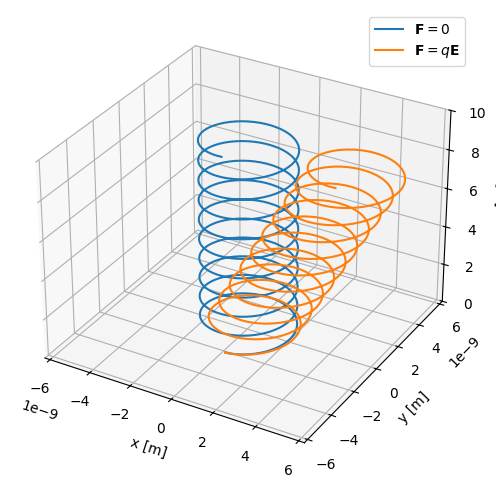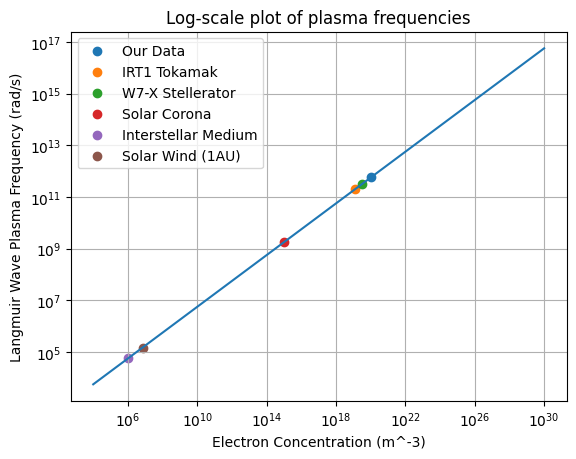# Frequency Plasma Parameters (plasmapy.formulary.frequencies)

Functions to calculate fundamental plasma frequency parameters.

## Functions

 gyrofrequency(B, particle[, signed, Z, to_hz]) Calculate the particle gyrofrequency in units of radians per second. lower_hybrid_frequency(B, n_i, ion[, to_hz]) Return the lower hybrid frequency. plasma_frequency(n, particle[, z_mean, to_hz]) Calculate the particle plasma frequency. upper_hybrid_frequency(B, n_e[, to_hz]) Return the upper hybrid frequency.

## Aliases

PlasmaPy provides aliases of the most common plasma functionality for user convenience. Aliases in PlasmaPy are denoted with a trailing underscore (e.g., alias_). For further details, please refer to the contributor guide’s section on aliases.

 oc_(B, particle[, signed, Z, to_hz]) Alias to gyrofrequency. wc_(B, particle[, signed, Z, to_hz]) Alias to gyrofrequency. wlh_(B, n_i, ion[, to_hz]) Alias to lower_hybrid_frequency. wp_(n, particle[, z_mean, to_hz]) Alias to plasma_frequency. wuh_(B, n_e[, to_hz]) Alias to upper_hybrid_frequency.

## Lite-Functions

Lite-functions are optimized versions of existing plasmapy functions that are intended for applications where computational efficiency matters most. Lite-functions accept numbers and NumPy arrays that are implicitly assumed to be in SI units, and do not accept Quantity objects as inputs. For further details, please refer to the contributor guide’s section on lite-functions.

Caution

Lite-functions do not include the safeguards that are included in most plasmapy.formulary functions. When using lite-functions, it is vital to double-check your implementation!

 plasma_frequency_lite(n, mass, z_mean[, to_hz])

## Examples